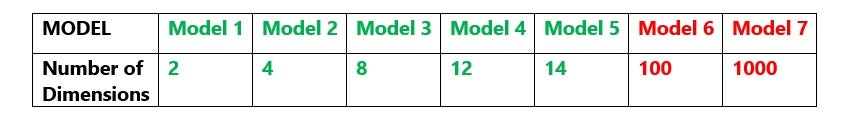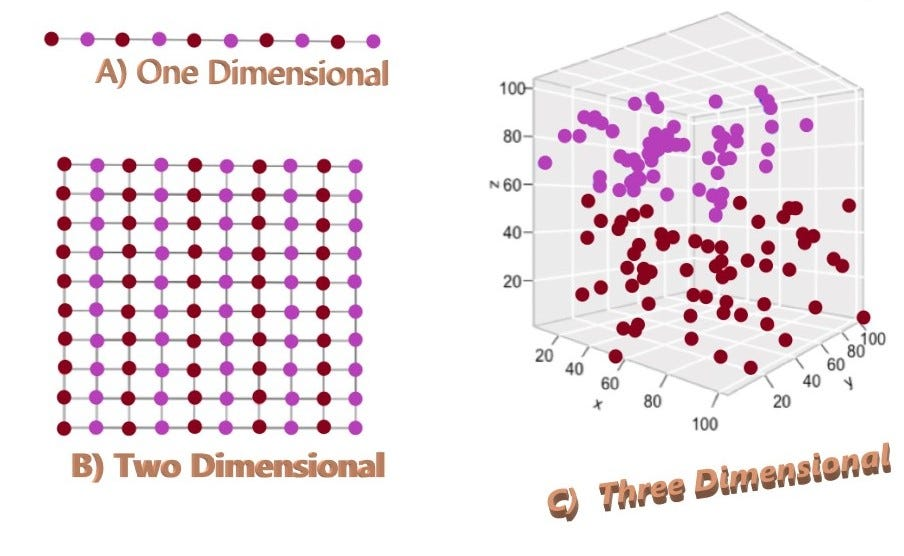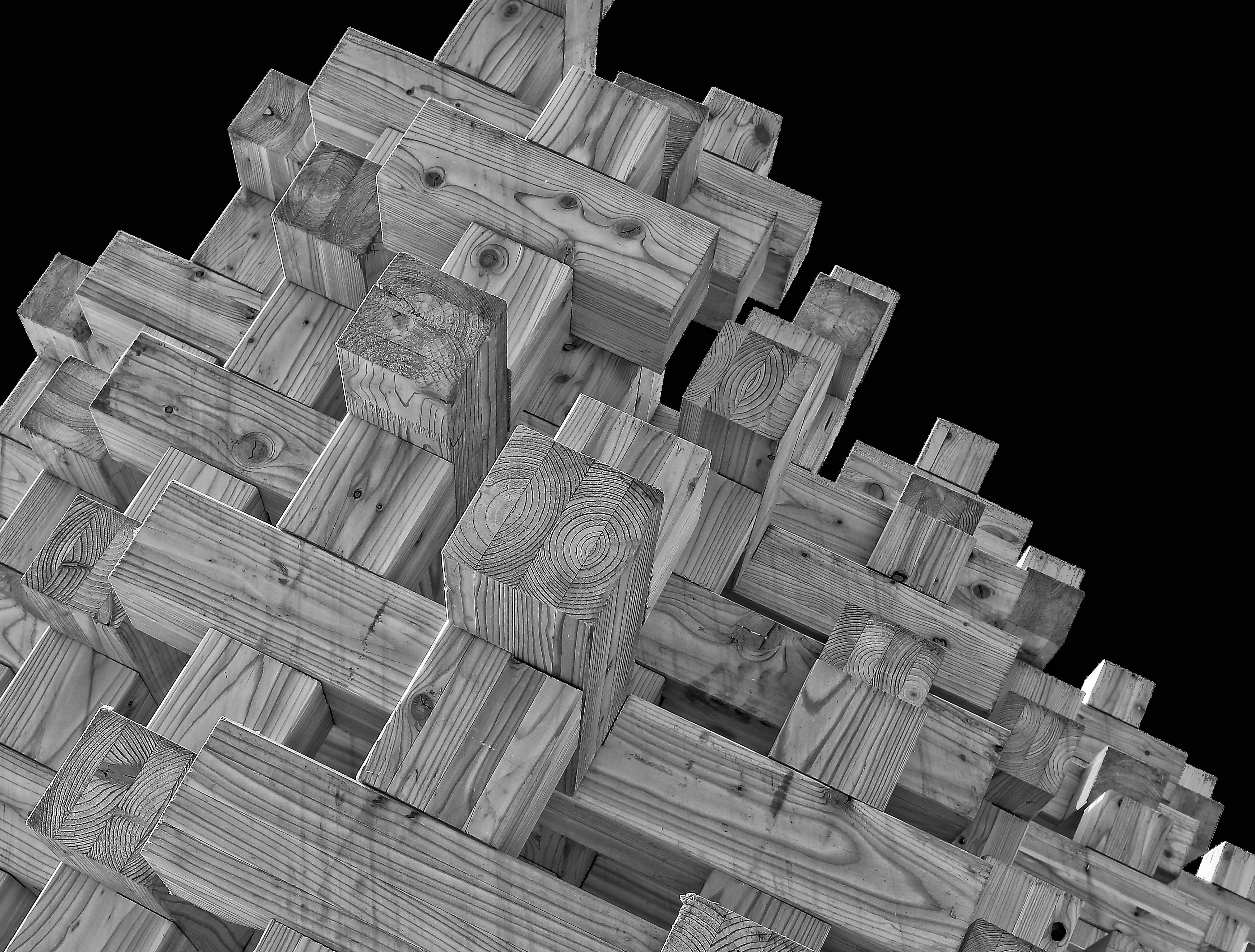1603358700

# Curse of Dimensionality

The Curse of Dimensionality — a catchy term is termed by mathematician Richard Bellman in his book “Dynamic Programming” in 1957 which refers to the fact that problems can get a lot harder to solve on high-dimensional instances.

**Dimension **in very simple terms means the attributes or features of a given dataset.

This sounds pretty simple. So why are we using such a negative word curse associated with dimensions? What is the **curse **here?

### Let us learn the curse of dimensionality with a general example.

If anybody asks me What is a Machine learning Model?

Speaking in layman terms, when we give the dataset for training and the output of the training phase is a Model.

Suppose we have 7 models each having a different number of dimensions keeping the motive of the model the same in all the 7 models:What we observe here is the number of features that we are giving to the training phase to generate the model is increasing exponentially.

So the question arises what is the relation between the number of dimensions and the model?

Can we say more number of features will result in a better model?

The answer is yes but …oh yes! there is a but here.

We can say that more number of features results in a better model but this is true only to a certain extent lets call that extent threshold.

#machine-learning

## Buddha Community1597737720

## k-Nearest Neighbors and the Curse of Dimensionality

### Creating your own k-Nearest Neighbor modelThere is always a trade off between things in life. If you take up a certain path then there is always a possibility that you might have to compromise with some other parameter. Machine Learning models are no different, considering the case of k-Nearest Neighbor there has always been a problem which has a huge impact over classifiers that rely on pairwise distance and that problem is nothing but the “Curse of Dimensionality”. By the end of this article you will be able to create your own k-Nearest Neighbor Model and observe the impact of increasing the dimension to fit a data set. Let’s dig in!

Creating a k-Nearest Neighbor model:

Right before we get our hands dirty with the technical part, we need to lay the buttress for our analysis, which is nothing but the libraries.

``````import matplotlib.pyplot as plt
import matplotlib.patches as patches

import numpy as np
import seaborn as sns
from scipy import stats
sns.set_style("white")

## for 3d plots
from ipywidgets import interact, fixed
from mpl_toolkits import mplot3d

from tqdm import tqdm

from sklearn.neighbors import KNeighborsClassifier
from sklearn.model_selection import validation_curve, train_test_split

plot_colors = np.array(sns.color_palette().as_hex())
view raw
knn_lib.py hosted with ❤ by GitHub
``````

Thanks to inbuilt machine learning packages which makes our job quite easy.

#data-science #curse-of-dimensionality #ds-in-the-real-world #data-visualization #machine-learning #data analysis1624928520

## Dimensional Modeling

A tutorial on the concepts and practice of Dimensional Modeling, the Kimball Method.

#modeling #dimensional #data warehousing1601130840

## The Curse of Dimensionality… minus the curse of jargon

The curse of dimensionality! What on earth is that? Besides being a prime example of shock-and-awe names in machine learning jargon (which often sound far fancier than they are), it’s a reference to the effect that adding more features has on your dataset. In a nutshell, the curse of dimensionality is all about loneliness.

In a nutshell, the curse of dimensionality is all about loneliness.

Before I explain myself, let’s get some basic jargon out of the way. What’s a feature? It’s the machine learning word for what other disciplines might call a predictor / (independent) variable / attribute / signal. Information about each datapoint, in other words. Here’s a jargon intro if none of those words felt familiar.

Data social distancing is easy: just add a dimension. But for some algorithms, you may find that this is a curse…

When a machine learning algorithm is sensitive to the curse of dimensionality, it means the algorithm works best when your datapoints are surrounded in space by their friends. The fewer friends they have around them in space, the worse things get. Let’s take a look.

#mathematics #machine-learning #editors-pick #artificial-intelligence #data-science1603358700

## Curse of Dimensionality

The Curse of Dimensionality — a catchy term is termed by mathematician Richard Bellman in his book “Dynamic Programming” in 1957 which refers to the fact that problems can get a lot harder to solve on high-dimensional instances.

**Dimension **in very simple terms means the attributes or features of a given dataset.

This sounds pretty simple. So why are we using such a negative word curse associated with dimensions? What is the **curse **here?

### Let us learn the curse of dimensionality with a general example.

If anybody asks me What is a Machine learning Model?

Speaking in layman terms, when we give the dataset for training and the output of the training phase is a Model.

Suppose we have 7 models each having a different number of dimensions keeping the motive of the model the same in all the 7 models:What we observe here is the number of features that we are giving to the training phase to generate the model is increasing exponentially.

So the question arises what is the relation between the number of dimensions and the model?

Can we say more number of features will result in a better model?

The answer is yes but …oh yes! there is a but here.

We can say that more number of features results in a better model but this is true only to a certain extent lets call that extent threshold.

#machine-learning1597741140

## Dimensionality Reduction

It is easy for us to visualize two or three dimensional data, but once it goes beyond three dimensions, it becomes much harder to see what high dimensional data looks like.

Today we are often in a situation that we need to analyze and find patterns on datasets with thousands or even millions of dimensions, which makes visualization a bit of a challenge. However, a tool that can definitely help us better understand the data is dimensionality reduction.

In this post, I will discuss t-SNE, a popular non-linear dimensionality reduction technique and how to implement it in Python using sklearn. The dataset I have chosen here is the popular MNIST dataset.

## Overview

T-Distributed Stochastic Neighbor Embedding, or t-SNE, is a machine learning algorithm and it is often used to embedding high dimensional data in a low dimensional space .

In simple terms, the approach of t-SNE can be broken down into two steps. The first step is to represent the high dimensional data by constructing a probability distribution P, where the probability of similar points being picked is high, whereas the probability of dissimilar points being picked is low. The second step is to create a low dimensional space with another probability distribution Q that preserves the property of P as close as possible.

In step 1, we compute the similarity between two data points using a conditional probability p. For example, the conditional probability of j given i represents that x_j would be picked by x_i as its neighbor assuming neighbors are picked in proportion to their probability density under a Gaussian distribution centered at _x_i _. In step 2, we let _y_i _and y_j to be the low dimensional counterparts of x_i and _x_j, _respectively. Then we consider q to be a similar conditional probability for y_j being picked by y_i and we employ a **student t-distribution **in the low dimension map. The locations of the low dimensional data points are determined by minimizing the Kullback–Leibler divergence of probability distribution P from Q.

#data-visualization #sklearn #dimensionality-reduction #data-science #machine-learning #data analysis